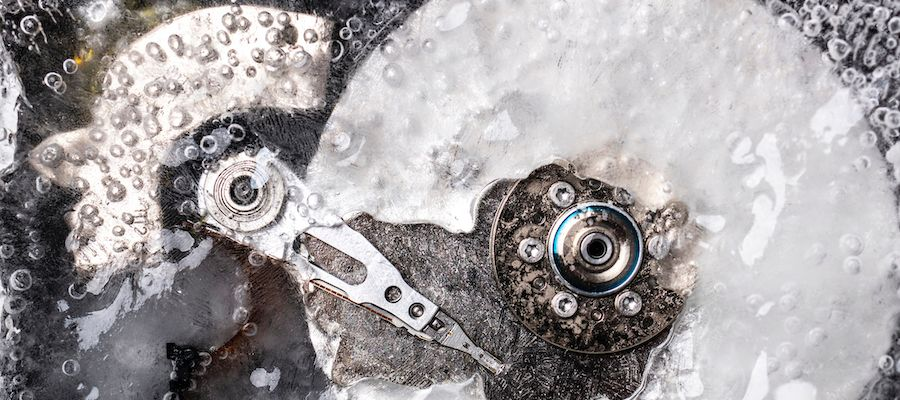# How much do you know about Material Science and Environmental Engineering?

## Test your skills in Material Science and Environmental Engineering. There is one good answer, or one that is better than the others. Do you get full points?### Quiz Questions

• 1.
Hygroscopy, it is an ability to:
• A.
Filter hazardous species
• B.
Assimilate moisture directly from the air
• C.
Measure the diameters of droplets
• D.
Fill up cylinders with acidic water
• 2.
The damping in the SDOF-system used for calculating the response spectrum of a shock is often given as a Q-factor of 10. What relative critical damping ratio does Q=10 correspond to?
• A.
10%
• B.
5%
• C.
4%
• D.
2%
• 3.
A metal usually reacts quickly with corrosion accelerators but then slowly the corrosion rate decreases, due to:
• A.
The metal ions are to heavily stuck in the metal matrix
• B.
Lack of light which must be present to increase the activation energy
• C.
A metal oxide is formed which passivates the surface
• D.
Electrons are hindered on their way from the surface
• 4.
A stationary random vibration is best described by:
• A.
A long time series of acceleration data
• B.
Amplitude spectrum with unit g
• C.
Power spectral density with unit g2/Hz
• D.
Energy spectral density with unit g2s/Hz
• 5.
Atmospheric corrosion is:
• A.
Corrosion in space
• B.
Corrosion taken place during high temperature
• C.
Corrosion in seawater
• D.
An electro-chemical process on a metal surface exposed to the atmospheric conditions
• 6.
What is the largest uncertainty source for material fatigue prediction error, when a large structure is verified for sinusoidal vibration resistance by calculation only?
• A.
Structural damping
• B.
Geometry in FE-model (e.g. notch radius)
• C.
Stress response calculation method
• D.
Fatigue prediction from given stress response history
• 7.
Best simulating the sun light, in order to make the most accurate WoM (UV-light) tests, are:
• A.
Xe-arc lamp
• B.
Fluorescent UV lamps
• C.
Carbon Arc burners
• D.
LED lamps
• 8.
Assuming fatigue as failure mode and a Wöhler slope of 5, what relative test duration (fraction) is sufficient for a damage-equivalent test if the PSD is multiplied with 2?
• A.
(1/5)^2 (or 1/25)
• B.
(1/2)^5 (or 1/32)
• C.
1/(2*5) (or 1/10)
• D.
(1/2)^(5/2) (approx. 1/5.7)

### Quiz Outcomes

• 1.
a great result
Great that you completed the quiz!Easy, or maybe very difficult?Make sure your knowledge is always up to date. One easy way is to buy the book “Environmental Engineering Handbook”, a complete handbook as reviews all the fundamentals and practices for material science and environmental engineering. Author: Swedish Environmental Engineering Society(Scroll down for result)